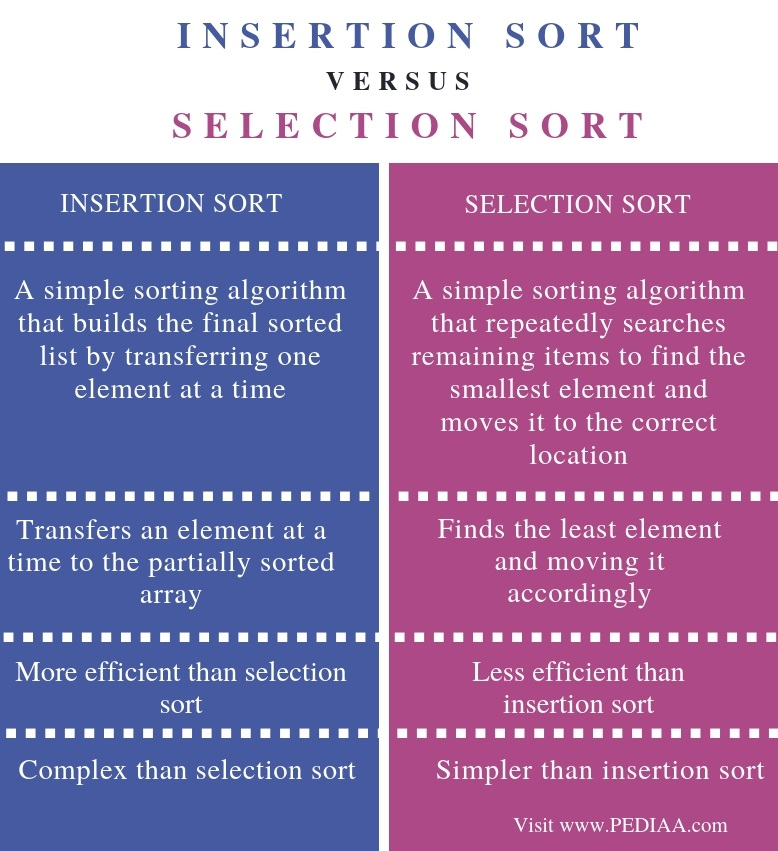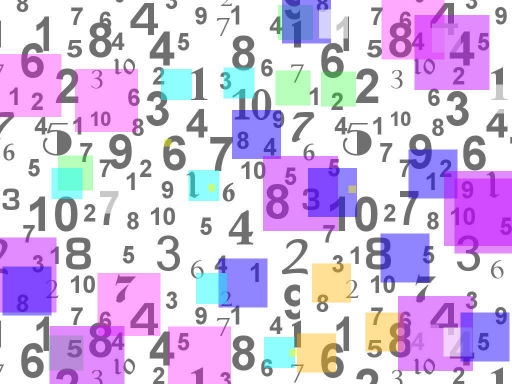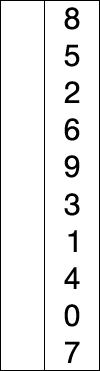# What is the Difference Between Insertion Sort and Selection Sort

The main difference between insertion sort and selection sort is that insertion sort performs sorting by exchanging an element at a time with the partially sorted array while selection sort performs sorting by selecting the smallest element from the remaining elements and exchanging it with the element in the correct location.

An algorithm is a sequence of steps to solve a problem. We use algorithms in computer programming to solve a problem. Moreover, sorting is an important operation performed on a set of data. There are various algorithms to sort a data set. Insertion sort and selection sort are two simple sorting algorithms.

### Key Areas Covered

1.  What is Insertion Sort
– Definition, Functionality
2. What is Selection Sort
– Definition, Functionality
3. Difference Between Insertion Sort and Selection Sort
– Comparison of key differences

### Key Terms

Insertion Sort, Selection Sort, Sorting Algorithms## What is Insertion Sort

Insertion sort algorithm performs sorting by transferring one element at a time to the partially sorted array.  An important feature of this algorithm is that it has a low overhead.Consider the following example.

20  100  3  25  6  95  45  55

We consider 20 is in the partially sorted array.

Consider 100. It is greater than 100.  20 and 100 are in the partially sorted array.

Now, consider 3.  As it is less than 20, we can place it in the correct position. Now 3, 20 and 100 are in the partially sorted array.

3  20   100  25  6  95  45  55

Now, let’s consider 25. It is less than 100 but greater than 20, so we can place it in the correct position. 3,20, 25,100 are now in the partially sorted array.

3  20  25  100  6  95  45 55

Let’s consider 6. It is greater than 3 but less than 20. So, we can place it in the correct position. 3,6,20,25,100 are in the partially sorted array.

3  6  20  25  100  95  45  55

Let’s consider 95. It is greater than 25 but less than 100. We can locate that element in the correct position.

3  6  20  25  95  100  45  55

Now, consider 45. It is greater than 25 but less than 95. So, we can place it in the correct position. 3, 6, 20, 25, 45, 95, 100 are in the partially sorted array.

3  6  20  25  45  95  100  55

Next, consider 55. It is greater than 45 but less than 95. Therefore, we can place it in the correct position.

3  6  20  25  45  55  95  100

Now, we can see that all the elements are sorted.

## What is Selection Sort

Selection sort performs sorting by selecting the smallest element from the remaining elements and placing it at the correct position.Consider the following example.

20  100  3  25  6  95  45  55

Here, the lowest element is 3. Therefore, we can exchange it with the element in the first position (which is 20).

3  100   20  25  6  95  45  55

The lowest element out of the remaining elements is 6. We can exchange it with the element in the second position (which is 100).

3   6   20  25  100  95  45  55

The smallest element out of the remaining elements is 20. It is already in the 3rd position. Thus, there is no need for moving the elements.

Next, the smallest element out of the remaining is 25. It also is in the 4th position, and there is no need for moving the elements.

Now the minimum element out of the remaining is 45. We can exchange it with the element in the 5th position (which is 100).

3  6  20  25  45  95 100  55

The minimum element out of the remaining numbers is 55. Therefore, we can exchange it with the element in the 6th position which is 95.

3  6  20  25  45  55 100  95

Now, the lowest element out of the remaining is 95. We can exchange it with the element in the 7th position, which is 100.

3  6  20  25  45  55  95  100

The remaining element is 100 and it is in the correct position. Now, we can see that the elements are sorted.

## Difference Between Insertion Sort and Selection Sort

### Definition

Insertion sort is a simple sorting algorithm that builds the final sorted list by transferring one element at a time. Selection sort, in contrast, is a simple sorting algorithm that repeatedly searches remaining items to find the smallest element and moves it to the correct location. Thus, this is the main difference between insertion sort and selection sort.

### Functionality

Insertion sort transfers an element at a time to the partially sorted array while selection sort finds the smallest element and move it accordingly.

### Efficiency

Another difference between insertion sort and selection sort is that the insertion sort is efficient than selection sort.

### Complexity

Complexity is also a difference between insertion sort and selection sort. Insertion sort is more complex than selection sort.

### Conclusion

Insertion sort and selection sort are two sorting algorithms. Both are suitable for sorting a small dataset. The main difference between insertion sort and selection sort is that insertion sort performs sorting by exchanging an element at a time with the partially sorted array while selection sort performs sorting by selecting the smallest element from the remaining elements and by exchanging it with the element in the correct location.

##### References:

1.“Insertion Sort.” Wikipedia, Wikimedia Foundation, 3 Feb. 2019, Available here.
2.“What Is an Insertion Sort? – Definition from Techopedia.” Techopedia.com, Available here.
3.”Selection Sort”, Available here.

##### Image Courtesy:

1.”Numbers” By The original uploader was Ianmacm at English Wikipedia. – graphic by member ianmacm (Public Domain) via Commons Wikimedia
2. “Selection-Sort-Animation” By Joestape89 (CC BY-SA 3.0) via Commons Wikimedia